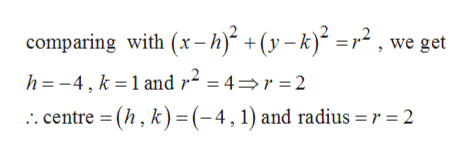# For Exercise, graph the equation.(x + 4)2 + ( y − 1)2 = 4

Question
1 views

For Exercise, graph the equation.

(x + 4)2 + ( y − 1)2 = 4

check_circle

Step 1

Given,

Step 2

Clearly it is an equation of a circle.

Now comparing the given...help_outlineImage Transcriptionclosecomparing with (x- h)* +(y – k)* =r² , we get h = -4, k = 1 and r² = 4=r = 2 .. centre = (h , k)=(-4,1) and radius = r = 2 %3D %3D fullscreen

### Want to see the full answer?

See Solution

#### Want to see this answer and more?

Solutions are written by subject experts who are available 24/7. Questions are typically answered within 1 hour.*

See Solution
*Response times may vary by subject and question.
Tagged in

### Other